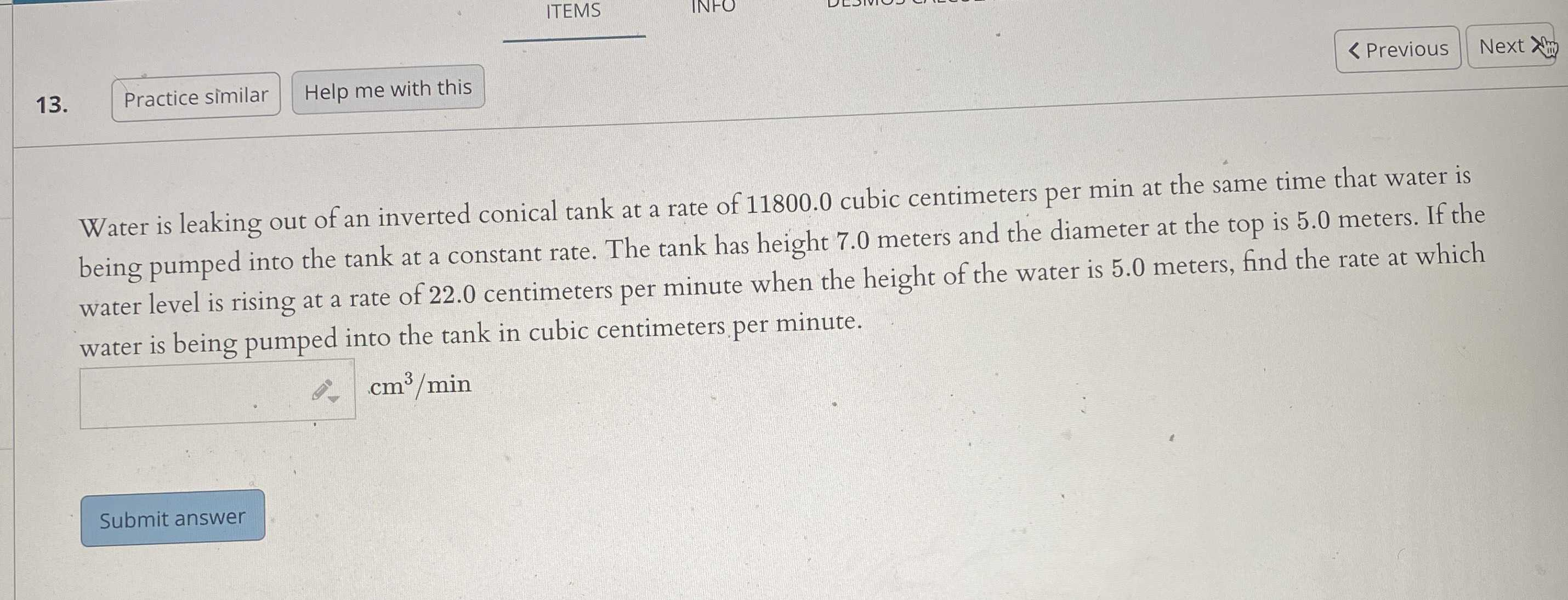### ¿Todavía tienes preguntas de matemáticas?

Pregunte a nuestros tutores expertos
Algebra
Pregunta13. Practice similar Help me with this Water is leaking out of an inverted conical tank at a rate of $$11800.0$$ cubic centimeters per min at the same time that water is being pumped into the tank at a constant rate. The tank has height $$7.0$$ meters and the diameter at the top is $$5.0$$ meters. If the water level is rising at a rate of $$22.0$$ centimeters per minute when the height of the water is $$5.0$$ meters, find the rate at which water is being pumped into the tank in cubic centimeters per minute.